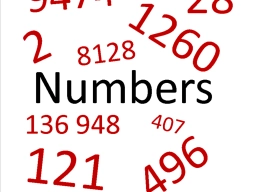# The difference 4

The difference between four times a number and five is the same as three more than twice a number. Find the number.

x =  4

### Step-by-step explanation:Did you find an error or inaccuracy? Feel free to write us. Thank you!

Tips for related online calculators
Do you have a linear equation or system of equations and looking for its solution? Or do you have a quadratic equation?